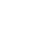# HDOJ1008：Elevator——简单数学题

Problem Description
The highest building in our city has only one elevator. A request list is made up with N positive numbers. The numbers denote at which floors the elevator will stop, in specified order. It costs 6 seconds to move the elevator up one floor, and 4 seconds to move down one floor. The elevator will stay for 5 seconds at each stop.

For a given request list, you are to compute the total time spent to fulfill the requests on the list. The elevator is on the 0th floor at the beginning and does not have to return to the ground floor when the requests are fulfilled.

Input
There are multiple test cases. Each case contains a positive integer N, followed by N positive numbers. All the numbers in the input are less than 100. A test case with N = 0 denotes the end of input. This test case is not to be processed.

Output
Print the total time on a single line for each test case.

Sample Input
1 2
3 2 3 1
0

Sample Output
17
41

Author
ZHENG, Jianqiang

3 1 1 1

21

```#include
int main()
{
int n;
while (~scanf("%d", &n)&&n!=0)
{
int f, fi,j, sum;
f = sum = 0;
for (j = 0; j f)
{
sum += 4 * (fi - f);
}
//if (j!=n-1)注意最后也要停五秒
sum += 5;
}
printf("%dn", sum);
}
return 0;
}
```

## 《HDOJ1008：Elevator——简单数学题》上有2条评论

1.匿名说道：

初来乍到，踩一下很有必要！

甲午年(马)冬月廿十 2015-1-10

2.老牛博客说道：

英文是我弱项。。。